Impact Factor 3.498 | CiteScore 3.3
More on impact ›

# Frontiers in Earth Science## ORIGINAL RESEARCH article

Front. Earth Sci., 05 July 2021 | https://doi.org/10.3389/feart.2021.686832

# A View for Atmospheric UnpredictabilityXiuhua Cai1,Hongxing Cao1*,Xiaoyi Fang1*,Jingli Sun2 andYing Yu1
• 1Engineering Center of Chinese Academy of Meteorological Sciences, Beijing, China
• 2CMA Public Meteorological Service Center, Beijing, China

Based on Chaotic Dynamics, this paper illustrated the necessity of research and the objective existence of atmospheric unpredictability. Actually, inaccurate forecast happens all the time in both operational weather forecasting and climate prediction in which atmospheric unpredictability hides. By means of Discrete Mathematics, this paper also defined the Degree of Hesitation and the Predictable Days with which to discuss and compare the relationship between the predictability and unpredictability of several different forecast objects. In addition, this paper discussed the approaches of evaluating the atmospheric predictability and unpredictability, emphatically showed the Experience Assessment Method. At the last, this paper also proved the existence of atmospheric unpredictability by an example.

## Introduction

The study of atmospheric predictability, that is weather predictability, and climate predictability, has had a long history. The earliest study of dynamical predictability appeared in 1957 when Thompson firstly raised the issue of the predictability of Numerical Weather Prediction. Suppose an error exists in the initial field, it will be doubled after a period of time the integral of the equation of atmospheric motion, and then it will get bigger and bigger. This length of time is called Predictability (Thompson, 1957). Lorenz further studied weather predictability and laid the theoretical foundation of dynamic predictability in 1963 (Lorenz 1963). Generally, the Predictability of daily weather forecasting is two weeks. Predictability was later extended to statistical predictability and statistical-dynamical predictability (Xin et al., 1988). Zheng et al. (2013) used similar-dynamical method to correct the forecast error of the predictable components, so as to statistically reduce the model error and the influence of the random components on the predictable components. The 1998 catastrophic floods of Nenjiang River and Songhuajiang River was accurately forecasted because some important signs were caught in time: In the winter of 1997, there were frequent heavy snowfalls on the Qinghai-Tibet Plateau; At the same time, the “El Niño phenomenon” occurred in China again and again. Chinese meteorologists carried out complicated calculations with several models, and finally recognized that there would be a big flood next year. Due to different definitions of predictability and different research methods, there were various predictability studies, thus the conclusions are often significantly different from each other (Wang, 2005; Li et al., 2006; Wang, 2009; Chen et al., 2019; Mu et al., 2020; Zhuang et al., 2020).

In 1963, Lorenz discovered the chaotic phenomenon in the simplified Thermal Convection Equations. He not only opened up the study of modern nonlinear dynamics, but also deepened the understanding of the predictability of weather and climate. In a chaotic system, the behavior of the system is very sensitive to the initial conditions, that is, as the initial conditions of the system slightly change, the system will reach a completely different state after enough time. Lorenz figuratively called the initial sensitivity as Butterfly Effect: a butterfly flapping its wings in Brazil may cause a tornado in Texas! According to Chaos Theory, even if a model is completely determined, even if the numerical solution is sufficiently accurate, the result may still be random after a long-time evolution. In other words, the reason that a dynamic system cannot forecast accurately for a long period of time is that the prediction accuracy is determined not only by external factors, but also by the internal dynamic characteristics and internal randomness of the system. The sensitivity of a dynamic system to initial conditions makes long-term prediction impossible. However, in a short period of time, the divergence of system motion orbit should be small, so it is feasible to use the observation data for short-term prediction. In short, deterministic systems are predictable in short term, but unpredictable in long term.

So far, an implicit subjective assumption in the study of predictability has always existed: since unpredictability and predictability are complementary and normalized, which means the sum of predictability and unpredictability is 1, then once predictability is determined, unpredictability would be naturally determined at the same time. Unfortunately, that is not the fact. Unpredictability is an objective reality and studying predictability alone can’t replace the study of unpredictability.

Firstly, unpredictability is closely related to time. As time goes on, the intensity of unpredictability changes. In a short period of time, unpredictability accounts for a small proportion, but in a long period of time, unpredictability accounts for an increasing proportion. This is an very important feature of unpredictability. The intensity and components of unpredictability are closely related to forecast period and timeliness. The longer the period, the bigger the unpredictability. The shorter the period, the more accurate prediction.

Secondly, both predictability and unpredictability have three key elements: the prediction equations or forecast model, the initial values, and the chaos in atmospheric change which cannot be expressed with equations. They are the three most important parameters to determine the accuracy of atmospheric predictability: 1) If the prediction equation is flawed, of course forecast will not be accurate; 2) Even if the prediction equation is defect-free, a small error in the initial value will be gradually enlarged and make the prediction accuracy worse and worse after a long period of integral time; 3) Even if the prediction equation is defect-free and the initial value is accurate, prediction accuracy still can’t be assured for the existence of atmospheric chaos. Essentially, chaos is caused by the unstable nature of the atmosphere itself, and is one of the laws of atmospheric motion. Since chaos cannot be described by any equation, the sudden change in atmospheric motion caused by chaos is also unpredictable. When chaos plays a leading role, the accuracy of the weather forecast is naturally impossible to talk about.

The atmosphere is an non-adiabatic and nonlinear chaotic system (Kong et al., 2008), thus the existence of its unpredictability is obvious. Palmer (1990) discussed the unpredictability of the atmosphere from the perspective of chaos dynamics, but he did not discuss the topic of unpredictability more.

Here we will re-understand the unpredictability of atmosphere, and discuss the relationship between predictability and unpredictability with the help of Discrete Mathematics, and then further explore the predictability of weather and climate.

Similar to the quantitative principles of the periphery theory (Cao, 1995; Cao et al., 2011; Niu et al., 2015; Cao, 2016; Yan et al., 2017), this paper will theoretically study the index of the unpredictability, and explore possible calculation methods.

## Study Methods

### Principle

Assume the Universe of discourse is X, and the variable $x∈X$, and the predictability $φ(x)$ and unpredicability $Ψ(x)$ satisfy:

$φ(x)∈[0,1]$
$Ψ(x)∈[0,1],$
$φ(x)+Ψ(x)≤1$
$ζ(x)=1−φ(x)−ψ(x).(1)$

ζ(x) in Eq. 1 is the degree of hesitation. Obviously, the degree of hesitation is not independent. Once research reduces hesitation, estimates of predictability or unpredictability will increase.

Define:

$α=(φα,ψα)(2)$

α in Eq. 2 is a predictor, $α+=(1,0)$ as a maximum predictor, and $α−=(0,1)$ as a minimum predictor.

Assume α = (φα, ψα) = (0.7, 0.2) the intuitive physical interpretation of this predictable number is: for a forecast object Y, suppose 10 people take part in making the forecast, 7 of them think they can predict it, 2 of them think they cannot, and 1 of them does not express their attitude. In other words, it's estimated that 70% of them can predict success, 20% of can’t, and 10% of them do not have any conclusions.

$μ(α)=φα+ψα(3)$
$V(α)=φα−ψα(4)$

μ(α) in Eq. 3 is a determinable function, and ν(α) in Eq. 4 is a function of success. Obviously, ν(α) bigger is better, and μ(α) bigger is not necessarily better.

Define

$β(x)=ς(x)φ(x)−ψ(x)(5)$

β(x) in Eq. 5 is the coefficient of hesitation. Obviously, the smallerβ(x), the better the predictability.

### Operational Rules

A predeterminable number is a binary number. As in Set Theory, it is defined by the following rules:

Assume the predeterminable number α = (φα, ψα), α1 = (φα1, ψα1), α2 = (φα2, ψα2), then.

1) complement operation

$αC=(ψα,φα)(6)$

2) union operation

$α1∪α2=(max{φα1,φα2}, min{ψα1,ψα2})(7)$

3) intersection operation

$α1∩α2=(max{φα1,φα2},max{ψα1,ψα2})(8)$

### Calculation Examples

To make it easier to understand the variables and numbers defined above, do the following example calculation.

Assume a weather station is going to model its precipitation and temperature in flood season with auto-regression, and then make a trial prediction report for future n years. Substituting the data into Eqs 1, Eqs 2, Eqs 4, the predictability and unpredictability of precipitation and temperature can be obtained. After normalization:

For precipitation.

R=(φR, ψR)= (0.6, 0.2)

Hesitation ζR = (1–0.6–0.2) = 0.2.

Success function ν R (α) = 0.6–0.2 = 0.4.

For temperature.

T= (φT, ψT)= (0.8, 0.1)

Hesitation ζT = (1–0.8–0.1) = 0.1.

Success function. $νT(α)=0.8−0.1=0.7$

The hesitating coefficients for precipitation and temperature are as follows:

β(R) = 0.2/(0.6–0.2) = 0.5

β(T) = 0.1/(0.8–0.1) = 0.143.

Thus, only comparing the single number, Hesitation coefficient, the predictability and unpredictability are comprehensively taken into account. In addition, we can see that the temperature forecast is much better than precipitation forecast.

Further substituting the data into Eqs 68, get the following:

The complement of the predictable precipitation. $αRC=(0.2,0.6)$

The complement of the predictable temperature. $ATC=(0.1,0.8)$

Union of predictable precipitation and predictable temperature

$αR∪​αT=(max(0.6,0.8),min(0.2,0.1))=(0.8,0.1)$

Intersection of predictable precipitation and predictable temperature

$αR∩​αT=(min(0.6,0.8),max(0.2,0.1))=(0.6,0.2)$

## Applications

Studying the unpredictability of daily weather is an important and practical topic. A lot of researches on the predictability of daily weather have been done (Xiong et al., 2000; Li et al., 2014; Dookie et al., 2019; Gao et al., 2019; Huang et al., 2019; Pan et al., 2019; Li et al., 2020), up to now the basic conclusion is that the predictable time limitation is two weeks. What is the unpredictable time limitation for daily weather? No one knows anything about it. This is due to the methodological problems in the study of predictability, that is, it is regarded as an unary problem, but in fact it is a binary problem. In practice, we also need to know the unpredictable time limitation length of the daily weather.

There are three ways to study the problem of atmospheric predictability:

1) Dynamics method: This method is a traditional research method. It uses atmospheric motion equation and analytically solves the simplified equation, or uses the numerical model to carry on the simulation computation, obtains the predictability and unpredictability of atmosphere;

2) Mathematical statistics method: this method uses regression model, multivariate analysis, and neural network and so on to establish the mathematical model to carry on the predictability research. Different results are often observed for using different models. As long as the predictability and unpredictability of atmosphere are defined in advance, both dynamic method and mathematical statistics method can obtain the valuable corresponding results. For example, the doubled forecast error is usually used as the predictability period of daily weather forecast. After deducted the error of average climatological field, the forecast is also used as the unpredictable period.

3) Empirical evaluation method: This method obtains the empirical valuations of predictability and unpredictability by assigning values to Fuzzy Delphi Method or Comprehensive Delphi Method. This method is very useful when there is only a few observations or it is difficult to determine by dynamic method or mathematical statistics method.

The operation of Delphi Method is that the organizer seeks multiple rounds of written opinions of experts anonymously by letters according to a pre-defined procedure of the system. Then, the organizer collates the opinions of each round and feeds it back to each expert as a reference, for their further analysis, assessments or prediction. This cycle will be repeated multiple times until the opinions of the experts basically converge (Jin and Wei, 2008). This method has a wide range of representativeness and good reliability. Many weather stations have experienced weathermen and it is appropriate for them to assess both predictability and unpredictability using Delphi Method. When multiple meteorological factors (independent variables) are used to predict a forecast object (dependent variables), the predictability and unpredictability can be obtained by using Fuzzy Comprehensive Evaluation Method. This requires to given Membership Grade in advance. Giving Membership Grade usually is empirical (Cao and Chen, 1988).

### A Case Study on Atmospheric Chaos

From the perspective of forecasting methods, to determine whether a certain kind of weather is about to occur is based on the analysis of whether the simulated future atmospheric conditions have the physical conditions for such weather. Once a kind of weather occurs, there must be some corresponding physical conditions, so we can explain and summarize afterward and help forecast in the future. However, these conditions are usually only necessary conditions rather than sufficient conditions for the occurrence of weather, in other words, even if there is the same condition, the same weather does not necessarily appear. This is due to the existence of chaos, the atmosphere can change suddenly, or be unstable. Although there is no problem with the model and the initial value, the prediction results are still inaccurate, resulting in the unpredictability of atmosphere. Moreover, the current limitation of human cognition may make the consideration of these conditions incomplete, which cause the unpredictability of atmosphere to be inevitable.

A failure case of Meiyu forecast in 2010: On June 15 and 16, the weather departments of Jiangsu, Shanghai and Zhejiang announced one after another that the Meiyu rainy season will start from June 17 according to the trend forecast of weather situation then. According to the weather data as of June 15, the Western Pacific Subtropical High Pressure (hereinafter referred to as Subtropical High) gradually moved northward, and the rainstorm area had covered Nanjing. However, there are too many uncertain factors in the weather system. Originally, the Subtropical High was expected to go northward and push the rain belt, but actually its position was very stable and stayed over Hunan, Jiangxi, Zhejiang, and Fujian in South China. According to the humidity field of 500 hPa (Figure 1) and 850 hPa (Figure 2) at 20:00 on the 17th, the ridge line of 500 hPa Subtropical High controls the South China continent at 18°N, 588 dagpm. At the same time, there are vortices (troughs) in North China, and Jiangsu, Shanghai, Zhejiang and other places are in the warm and humid air flow between the trough and the subtropical high, while the southwest low-level jet transfers water vapor at 850 hPa. The warm and humid tongue reaches the north of Anhui-Jiangsu and the shear line is located in western Anhui. All these physical conditions are very suitable for the occurrence of Meiyu. However, due to the existence of chaos, the weather suddenly changed: the North China vortex is falling southwards, the Subtropical High and 588 dagpm are pulling back, and the 850 hPa shear line is rapidly moving through Jiangsu and other places. Finally there is no occurrence of heavy rainfall which leads to the failure of Meiyu forecast. The unpredictability of the atmosphere is confirmed from one side.

FIGURE 1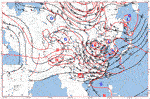FIGURE 1. Wind field, height field (black isolines) and temperature field (red isolines) at 500 hPa at 20:00 on June 17, 2010. (G. D, N, and L are high pressure center, low pressure center, warm center, and cold center in turn).

FIGURE 2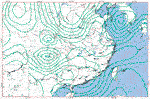FIGURE 2. Wind field and pseudo equivalent temperature field of 850 hPa at 20:00 on June 17, 2010 (green isolines).

### A Case Study on Forecast Timeliness

About the timeliness of weather forecast, a basic conclusion is that the predictability of a three-day forecast is very different from that of 15-day forecasts.

The predictability of a three-day forecast is relatively high. An example is the forecast of the heavy rainfall process in Jianghuai region between July 10th and 12th, 2010, according to the 500 hPa situation, the 850 hPa wind field, and the humidity field changes from July 9th to 10th. Comparing Figures 3, 4, we found that the eastern trough of the plateau was strengthening and moving eastward from 20:00 on July 9 to 08:00 on July 10, while the ridge line of the Subtropical High and 588 dagpm were rising northward. The corresponding 850 hPa southwest low-level jet and wet tongue was strengthening, too. At the same time, the warm shear line stayed in the Jianghuai region (Figures 5, 6). Therefore, there was a strong rainfall in the Jianghuai region from 10 to 12.

FIGURE 3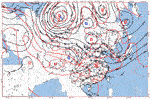FIGURE 3. Wind field, height field (black isolines) and temperature field (red isolines) at 500 hPa at 20:00 on July 9, 2010. (G. D, N and L are high pressure center, low pressure center, warm center and cold center in turn)

FIGURE 4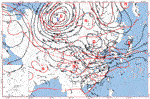FIGURE 4. Wind field, height field (black isolines) and temperature field (red isolines) at 500 hPa at 08:00 on July 10, 2010. (G. D, N and L are high pressure center, low pressure center, warm center and cold center in turn)

FIGURE 5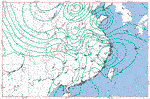FIGURE 5. Wind field and pseudo equivalent temperature field of 850 hPa at 20:00 on July 9, 2010 (green isolines).

FIGURE 6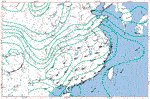FIGURE 6. Wind field and pseudo equivalent temperature field of 850 hPa at 08:00 on July 10, 2010 (green isolines).

The predictability of 15-day forecast is very low. For example, it is almost impossible to forecast the rainstorm process above in Jianghuai region occurred between July 10th to 12th according the weather situation on June 17. The reason is that due to the existence of chaos, the atmosphere may have unexpected abrupt changes.

## Conclusion

This paper has studied the unpredictability of atmosphere, based on defining some variables using discrete mathematics and periphery theory. These variables, such as the degree of hesitation and the numeric value of predictability, give us new ideas and mathematical expressions about both predictability and unpredictability of atmosphere. The unpredictability and the predictability are listed as the same unary, which allows us to make conceptual and methodological changes in the study of predictable problems, that is, whether using dynamics or statistics to study the problem of predictability, we should consider studying both predictability and unpredictability, instead of just studying predictability. Defining and discussing both of them at the same time move the predictability research onto a higher stage. Obviously, this paper is just an initial research on atmospheric predictability and unpredictability. Further, it is necessary to research more corresponding calculation using meteorological data and carrying out analysis of the variables. The study of unpredictability may open up a new field in atmospheric forecast.

## Discussion

The Short-term weather, that is, the weather in future 12–48 h, can be forecasted by using weather map forecasting method, statistical forecasting method, numerical forecasting method, etc., and the forecasting accuracy rate is relatively high. However, in operational weather forecasts, users often require daily forecasts from two weeks to one month, such as making a daily drill schedule for next month for a military drill, or preparing an outdoor meeting held 10 days later, or scheduling a travel three weeks later, etc. In these cases, two-week or one-month weather forecasts are demanded in advance. Usually, these demands are beyond the possibility of weather forecasts (Cao, 1983). Examples are as follows.

According to news reports on June 15, 2010 (Sun, 2010), the Jiangsu Meteorological Station (JMS) and the Nanjing Meteorological Administration (NMA) officially announced that Nanjing would enter the Meiyu rainy season next day and Nanjing citizens prepared for the rain. However, the heavy rain forecasted did not appear on schedule. Facing the query of netizen and citizen, the NMA publicly admitted the failure on its official website on June 23. As far as we know, this has been the first time that a meteorological department frankly admitted failure in weather forecasts in recent years.

From a scientific point of view, what can weather forecast do? What kind of weather can be predicted? What kind of weather cannot be predicted? The predictability of weather is closely related to weather type, scale of time and space as well as forecast time. Here we take the 1–3 days short-term forecast in advance as an example.

For rainfall forecast, a large-scale one can be predicted, such as “there will be a heavy rainfall process with moderate to heavy rain in North and Northeast China in the coming three days;” A medium to large-scale one basically can be predicted, such as “The strongest rainfall will occur tomorrow night to the day after tomorrow;” A medium to small-scale one basically cannot be predicted, such as “the rainfall of Beijing station from 02:00 to 03:00 tomorrow night will be 10 mm”. If the medium to small-scale rainfall is a scattered thunderstorm, it will be completely unpredictable. The larger the forecast time scale, the larger the predictable spatial scale; the smaller the forecast time scale, the smaller the predictable spatial scale.

For gale forecast, a large-scale one can be predicted, such as strong cold air processes, strong extratropical cyclones and strong tropical cyclones, but gale predictability for a specific time and a specific site is very low; A mesoscale wind, such as thunderstorm and gale, is predictable for an area but a location point; A small-scale wind is unpredictable, for example, it's impossible to predict a dust tornado in advance.

For snow forecast, the greater the scale, the higher the forecast reliability, such as cold wave and blizzard processes. Again, the difficulty of snow forecast will increase tremendously for a specific time and a specific site; The forecasts reliability of rain-snow conversion and freezing rain are lower than that of snowfall process only.

For temperature forecast, it is very reliable for an area forecast, but there are too many affecting factors for a specific site. For example, in the hot summer, people may feel that the temperature is above 40°C, but the weatherman says that it is only 35°C. The reason is that people is talking about the somatosensory temperature which is determined by the heat exchange between the body surface and the outside world, and is affected by sunshine, wind, humidity, and crow density, etc.

For climate prediction, what government and related users most concerned is next year’s harvest. That means to accurately forecast the precipitation, temperature of next year, especially of the flood season. Actually, fine climate predictions for more than one year or even for one season have exceeded the current achievable level of climate predictions. Behind all those problems, including it is too difficult to forecast and forecast accuracy is too low, the atmospheric unpredictability hides. With sufficiently study of the atmospheric unpredictability, we can do better in our meteorological services. We can reasonably explain the difficulty of a forecast project to the public or to the department decision-makers. We can even politely reject some forecast projects that actually are not possible.

## Data Availability Statement

The data analyzed in this study is subject to the following licenses/restrictions: The MICAPS dataset can only be accessed from inside China Meteorological Administration. Requests to access these datasets should be directed to Xiuhua Cai, caixha@126.com.

## Author Contributions

Conceptualization and methodology, XC and HC; Formal analysis, investigation, and writing—original draft preparation XC, HC, and XF; Thesis revision, JS; Editing, YY. All authors have read and agreed to the published version of the article.

## Funding

This research was funded by the Basic Research Fund (2021Z001) of Chinese Academy Meteorological Sciences.

## Conflict of Interest

The authors declare that the research was conducted in the absence of any commercial or financial relationships that could be construed as a potential conflict of interest.

## Acknowledgments

In the process of revising this paper, we have received the strong guidance from academician Chen Lianshou researcher Wang Yafei, researcher Ding Minghu, of Chinese Academy of Meteorological Sciences, national famous meteorological teacher Xiong Qiufen of China Meteorological Administration Training Center, and chief forecaster Zhang Tao of National Meteorological Center!

## References

Cao, H. X., and Chen, G. F. (1988). Fuzzy Set Method and its Application in Meteorology. Beijing: Meteorology Press.

Cao, H. X. (1983). Data Analysis Method of Local Weather Forecast. Beijing: Meteorological Press.

Cao, H. X., Feng, G. L., Cai, X. H., and Xia, C. H. (2011). Essentials of periphery(Jieke) Theory and its Application. Beijing: Science Press. doi:10.1063/1.3651831

CrossRef Full Text

Cao, H. X. (1995). Modelling of a System Boundary. Kybernetes 24 (6), 44–49. doi:10.1108/03684929510094299

Cao, Y. H. X. (2016). Modeling of System Boundary—Periphery Theory. Staarbruecken, Germany: Lambert Academic Publishing. doi:10.1109/radar.2016.7485284

CrossRef Full Text

Chen, F. J., Chen, J., and Wei, Q., (2019). A New Verification Method for Heavy Rainfall Forecast Based on predictabilityⅡ:Verification Method and Test. Acta Meteorologica Sinica77 (1), 28–42.

Dookie, N., Chadee, X. T., and Clarke, R. M. (2019). Trends in Extreme Temperature and Precipitation Indices for the Caribbean Small Islands: Trinidad and Tobago:Trinidad and Tobago. Theor. Appl. Climatol 136 (1/2), 31–44. doi:10.1007/s00704-018-2463-z

Gao, L., Chen, J., and Zheng, J. W., (2019). Progress in Researches on Ensemble Forecasting of Extreme Weather Based on Numerical Models. Adv. Earth Sci. 34 (7), 706–716.

Huang, Y., Jin, L., and Lu, H., (2019). A Combined Qualitative and Quantitative Prediction Scheme for Cold-Wet Extreme Weather in Guangxi Based on Intelligent Computing. Chin. J. Atmos. Sci. (in Chinese) 43 (6), 1424–1440.

Jin, J. L., and Wei, Y. M. (2008). Generalized Intelligent Evaluation Method for Complex Systems and its Application. Beijing: Science Press.

Kong, Y. S. H., Ji, L. L., Wang, Ch. Y., Liguo, Li, Liming, Z, et al. (2008). A New Technique of Non-linear Statistic Prediction and its Application in Atmospheric Systems. Kybernetes 37 (9/10), 1417–1424. doi:10.1108/k.2008.06737iab.001

Li, J. P., Ding, R. Q., and Chen, B. H. (2006). Review and Prospect of Atmospheric Predictability, Atmospheric Science Frontiers and Prospects in the Early 21st century. Beijing: Earth Science Department of NSFCMeteorological Press, 96–103.

Li, M., Zhang, S., Wu, L., Lin, X., Chang, P., Danabasoglu, G., et al. (2020). A High-Resolution Asia-Pacific Regional Coupled Prediction System with Dynamically Downscaling Coupled Data Assimilation. Sci. Bull. 65 (21), 1849–1858. doi:10.1016/j.scib.2020.07.022

Li, W., Liu, J., Chen, L., Zhang, P., and Ren, H. (2014). Spatiotemporal Distribution and Decadal Change of the Monthly Temperature Predictability Limit in China. Chin. Sci. Bull. 59, 4864–4872. doi:10.1007/s11434-014-0502-4

Lorenz, E. N. (1963). Deterministic Nonperiodic Flow. J. Atmos. Sci. 20, 130–141. doi:10.1175/1520-0469(1963)020<0130:dnf>2.0.co;2

Mu, M., Li, J. P., and Chou, J. F.,(2020). Theoretical Research on The Predictability of Climate System. Climatic Environ. Res. 7 (2), 227–235.

Niu, J. Q., Cao, H. X., Niu, B. S. H., and Wu, Y. P. (2015). Study of Atmospheric Predictability Based on Periphery Theory. Meteorology Environ. Res. 6 (3), 1–4.

Palmer, T. (1990). Views on the Unpredictability of Weather. Meteorol. Sci. Technology (5), 3–9.

Pan, X., Wang, G., and Yang, P. (2019). Introducing Driving-Force Information Increases the Predictability of the North Atlantic Oscillation. Atmos. Oceanic Sci. Lett. 12 (5), 329–336. doi:10.1080/16742834.2019.1628608

Sun, Y. L. (2010). Meiyu" No Rain? Weather Bureau: The Forecast Is Wrong! Modern Express. June 24, 2010).

Thompson, P. D. (1957). Uncertainty of Initial State as a Factor in the Predictability of Large-Scale Atmospheric Flow Pattern. Tellus (9), 275–295. doi:10.1111/j.2153-3490.1957.tb01885.x

Wang, H. J. (2005). Preliminary Research on the Inner-Atmosphere-Originated Seasonal Climate Predictability. Chin. J. Atmos. Sci. 29 (1), 64–70.

Wang, Y. (2009). How Do Outer Spiral Rainbands Affect Tropical Cyclone Structure and Intensity?*. * J. Atmos. Sci. 66, 1250–1273. doi:10.1175/2008JAS2737.1

Xin, Sh. T., Trans, Q. G., and Shengchang Hu, S. H. C. H. (1988). Predictability of Weather. Beijing: Meteorological Press.

Xiong, Q. F., Wang, L., and Zheng, Q. S., (2020). The Forecast Methods of Area Rainfall in the Sanxia Region and Experimental Impact Analyses. Meteorol. Monthly 26 (11), 19–23.

Yan, P. C. H., Wei, H., Cao, H. X., Jin, H, and Zhao, Y (2017). Research on System Control Based on a Novel Theory. Asia-Pacific Computer Science and Application Conference, 2017 March 11-12. Shanghai. ID, CSAC6518.

Zheng, Z., Huang, J., Feng, G., and Chou, J. (2013). Forecast Scheme and Strategy for Extended-Range Predictable Components. Sci. China Earth Sci. 56 (4), 878–889. doi:10.1007/s11430-012-4513-1

Zhuang, X., Min, J., Zhang, L., Wang, S., Wu, N., and Zhu, H. (2020). Insights into Convective-Scale Predictability in East China: Error Growth Dynamics and Associated Impact on Precipitation of Warm-Season Convective Events. Adv. Atmos. Sci. 37 (8), 893–911. doi:10.1007/s00376-020-9269-5

Keywords: atmospheric predictability, unpredictability, predictable days, chaos, periphery theory

Citation: Cai X, Cao H, Fang X, Sun J and Yu Y (2021) A View for Atmospheric Unpredictability. Front. Earth Sci. 9:686832. doi: 10.3389/feart.2021.686832

Received: 28 March 2021; Accepted: 18 June 2021;
Published: 05 July 2021.

Edited by:

Gert-Jan Steeneveld, Wageningen University and Research, Netherlands

Reviewed by:

Xiaojing Jia, Zhejiang University, China
Chenghai Wang, Lanzhou University, China

Copyright © 2021 Cai, Cao, Fang, Sun and Yu. This is an open-access article distributed under the terms of the Creative Commons Attribution License (CC BY). The use, distribution or reproduction in other forums is permitted, provided the original author(s) and the copyright owner(s) are credited and that the original publication in this journal is cited, in accordance with accepted academic practice. No use, distribution or reproduction is permitted which does not comply with these terms.

*Correspondence: Hongxing Cao, caohx1937@126.com; Xiaoyi Fang, xyfbj1977@126.com